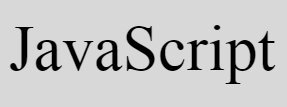# JavaScript Program to Check Even or Odd Number

« JavaScript Tutorial CodesCracker »

In this article, you will learn and get to check whether a number is an even or an odd number with and without using user-input in JavaScript. Here is the list of JavaScript program to check even or odd number:

• Check Even or Odd without User input
• Receive number from user, check and print whether the number is an even or odd. Live output of this program is also provided

## Check Even or Odd Number in JavaScript

This program checks whether the value stored in num variable is an even or an odd number. The document.write() method writes the data to an HTML output.

```<!doctype html>
<html>
<body>
<script>
var num=6;
if(num%2==0)
document.write(num + " is an Even Number");
else
document.write(num + " is an Odd Number");
</script>
</body>
</html>```

Save this code in a file with .html extension. Now open the file in a web browser. Here is the output:

If you change the value of num with 7 and save the code in same file. Then here is the output after refreshing or re-opening the file in a web browser:

### Allow User to Enter the Number

This is the actual program to check whether a number entered by user is an even or an odd number in JavaScript. This program receives input from user using TextBox.

```<!doctype html>
<html>
<script>
var num, temp;
function fun()
{
num = parseInt(document.getElementById("num").value);
if(num)
{
temp = document.getElementById("resPara");
temp.style.display = "block";
if(num%2==0)
document.getElementById("res").innerHTML = "Even";
else
document.getElementById("res").innerHTML = "Odd";
}
}
</script>
<body>

<p>Enter the Number: <input id="num"><button onclick="fun()">Check</button></p>
<p id="resPara" style="display:none;">It is an <span id="res"></span> Number</p>

</body>
</html>```

Here is its sample output:

Now enter any number say 17 and click on Check button to print a message that tells whether the entered number is an even or an odd number as shown in the snapshot of final output given below:

The following code:

`style="display:none;"`

is a CSS code, that hides an HTML element where it is written. Because it is written inside a P (paragraph) tag, whose id is resPara. So this paragraph gets hidden initially.

When user clicks on Check button, a function named fun() gets called. All the statements of this function gets executed. The following JavaScript statement:

`num = parseInt(document.getElementById("num").value);`

states that, the int (integer) value of an HTML element whose id is num gets initialized to num variable. And the statement given below:

`temp.style.display = "block";`

states that, an HTML element whose id is stored in temp, gets visible after executing this JavaScript statement. And the following JavaScript statement:

`document.getElementById("res").innerHTML = "Even";`

states that, the text Even gets written to an HTML element whose id is res.

#### Live Output of Previous Program

Here is the live output produced by previous JavaScript program on checking even or odd number:

Enter the Number:

JavaScript Online Test

« JavaScript Tutorial CodesCracker »